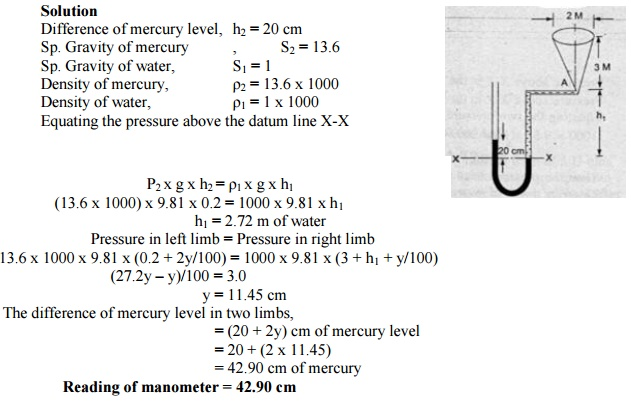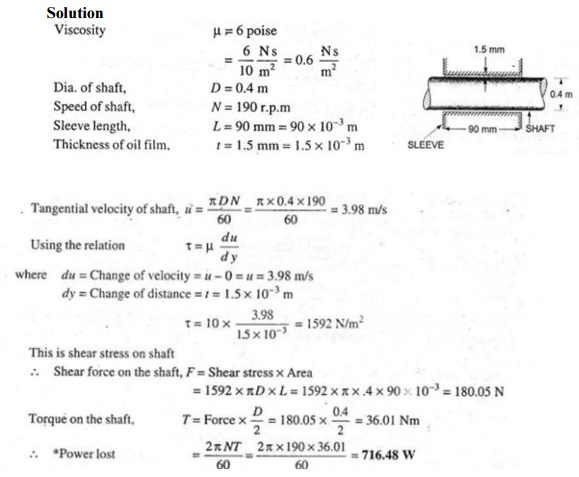Home | | Mechanics of Fluids | Problems: Civil - Fluid Properties And Fluid Statics

# Problems: Civil - Fluid Properties And Fluid Statics

Civil - Mechanics Of Fluids - Fluid Properties And Fluid Statics

1.What are the gauge pressure and absolute pressure at a point 3 m below the free surface of a liquid having a density of 1.53x103 kg/m3 if the atmospheric pressure is equivalent to 750 mm of mercury? The specific gravity of mercury is 13.6 and density of water is 1000 kg/m3.2.Figure shows a conical vessel having its outlet at A to which a U-tube manometer is connected. The reading of the manometer given in the figure shows when the vessel is empty. Find the reading of the manometer when the vessel is completely filled with water.3.Determine the total pressure on a circular plate of diameter 1.5 m which is placed vertically in water in such a way that the center of the plate is 3 m below the free surface of water. Find the position of centre of pressure also.4.A vertical sluice gate is used to cover an opening in a dam  wide and 1.2 m high. On the upstream of the gate, the liquid of Sp.gr 1.45 lies upto a height of 1.5 m above the top of the gate, whereas on the downstream side the water is available upto a height touching the top of the gate. Find the resultant force acting on the gate and position of centre of pressure. Find also the force acting horizontally at the top of the gate which is capable of opening it. Assume that the gate is hinged at the bottom.5.A block of wood of specific gravity 0.7 floats in water. Determine the meta-centric height of the block if its size is 2 m x 1 m x 0.8 m.6.Calculate the dynamic viscosity of oil which is used for lubrication between a square plate of size 0.8 mx0.8 m and an inclined angle of inclination 30 as shown in figure. The weight of the square plate is 300 N and it slides down the inclined plane with a uniform velocity of 0.3 m/s. The thickness of the oil film is 1.5 mm.7.Find the kinematic viscosity of an oil having density 981 kg/m. The shear stress at a point in oil is 0.2452 N/m and velocity gradient at that point is 0.2 per second.8.The dynamic viscosity of oil used for lubrication between a shaft and sleeve is 6 poise. The shaft is of diameter 0.4 m and rotates at 190 rpm. Calculate the power lost in the bearing for a sleeve length of 90 mm. The thickness of the oil film is 1.5 mm.9.If the velocity profile of a fluid over a plate is a parabolic with the vertex 20 cm from the plate, where the velocity is 120 cm/sec. calculate the velocity gradients and shear stresses at a distance of 0, 10, 20 cm from the plate, if the viscosity of the fluid is 8.5 poise.10. What is the bulk modulus of elasticity of a liquid which is compressed in a cylinder from a volume of 0.0125 m3 at 80 N/cm2 pressure to a volume of 0.0124 m3 at 150 N/cm2 pressure?11.The pressure outside the droplet of water of diameter 0.04 mm is 10.32 N/cm2 (atmospheric pressure). Calculate the pressure within the droplet if surface tension is given as 0.0725 N/m of water.12.Calculate the capillary rise in a glass tube of 2.5 mm diameter when immersed vertically in (a) water and (b) mercury. Take surface tension is 0.0725 N/m for water and 0.52 N/m for mercury in contact with air. The specific gravity for mercury is given as 13.6 and angle of contact is 130 o .13.Find out the minimum size of glass tube that can be used to measure water level if the capillary rise in the tube is to be restricted to 2 mm. Consider surface tension of water in contact with air as 0.073575 N/m.Study Material, Lecturing Notes, Assignment, Reference, Wiki description explanation, brief detail
Civil : Mechanics Of Fluids : Fluid Properties And Fluid Statics : Problems: Civil - Fluid Properties And Fluid Statics |